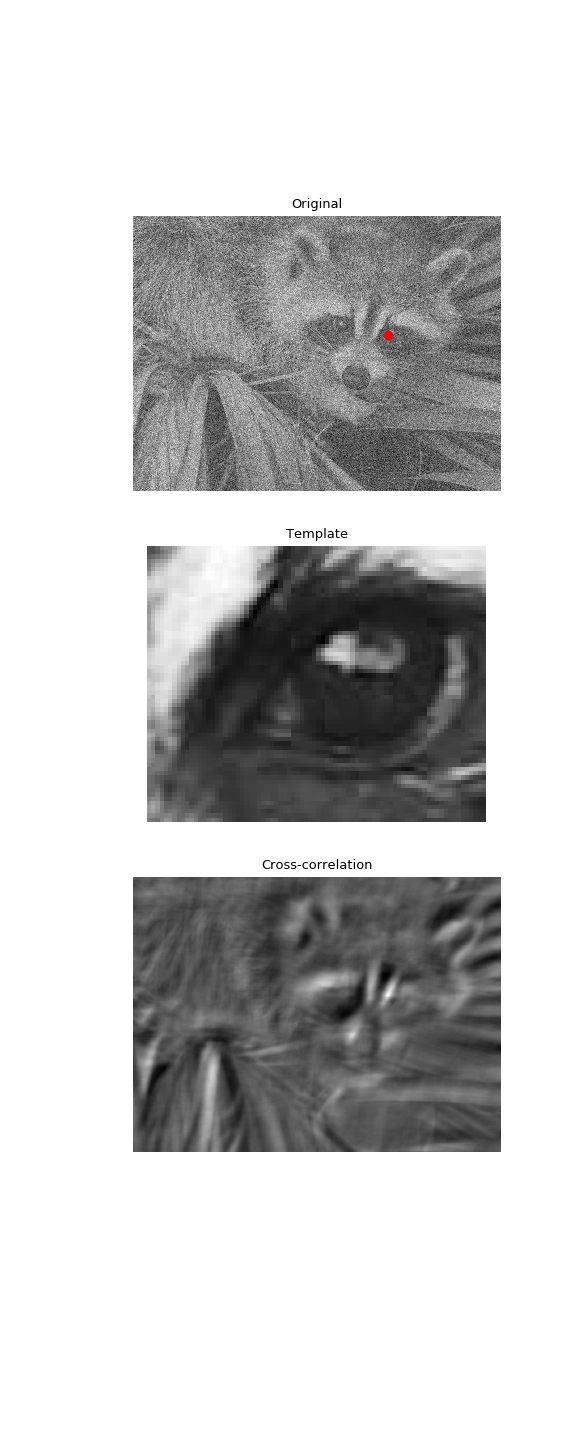# scipy.signal.correlate2d¶

scipy.signal.correlate2d(in1, in2, mode='full', boundary='fill', fillvalue=0)[source]

Cross-correlate two 2-dimensional arrays.

Cross correlate in1 and in2 with output size determined by mode, and boundary conditions determined by boundary and fillvalue.

Parameters
in1array_like

First input.

in2array_like

Second input. Should have the same number of dimensions as in1.

modestr {‘full’, ‘valid’, ‘same’}, optional

A string indicating the size of the output:

full

The output is the full discrete linear cross-correlation of the inputs. (Default)

valid

The output consists only of those elements that do not rely on the zero-padding. In ‘valid’ mode, either in1 or in2 must be at least as large as the other in every dimension.

same

The output is the same size as in1, centered with respect to the ‘full’ output.

boundarystr {‘fill’, ‘wrap’, ‘symm’}, optional

A flag indicating how to handle boundaries:

fill

pad input arrays with fillvalue. (default)

wrap

circular boundary conditions.

symm

symmetrical boundary conditions.

fillvaluescalar, optional

Value to fill pad input arrays with. Default is 0.

Returns
correlate2dndarray

A 2-dimensional array containing a subset of the discrete linear cross-correlation of in1 with in2.

Examples

Use 2D cross-correlation to find the location of a template in a noisy image:

>>> from scipy import signal
>>> from scipy import misc
>>> face = misc.face(gray=True) - misc.face(gray=True).mean()
>>> template = np.copy(face[300:365, 670:750])  # right eye
>>> template -= template.mean()
>>> face = face + np.random.randn(*face.shape) * 50  # add noise
>>> corr = signal.correlate2d(face, template, boundary='symm', mode='same')
>>> y, x = np.unravel_index(np.argmax(corr), corr.shape)  # find the match

>>> import matplotlib.pyplot as plt
>>> fig, (ax_orig, ax_template, ax_corr) = plt.subplots(3, 1,
...                                                     figsize=(6, 15))
>>> ax_orig.imshow(face, cmap='gray')
>>> ax_orig.set_title('Original')
>>> ax_orig.set_axis_off()
>>> ax_template.imshow(template, cmap='gray')
>>> ax_template.set_title('Template')
>>> ax_template.set_axis_off()
>>> ax_corr.imshow(corr, cmap='gray')
>>> ax_corr.set_title('Cross-correlation')
>>> ax_corr.set_axis_off()
>>> ax_orig.plot(x, y, 'ro')
>>> fig.show()#### Previous topic

scipy.signal.convolve2d

#### Next topic

scipy.signal.sepfir2d